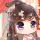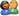﻿﻿﻿﻿﻿﻿﻿﻿﻿ [求助] Maxsumpath in 2D array_Android, Python及开发编程讨论区_Weblogic技术|Tuxedo技术|中间件技术|Oracle论坛|JAVA论坛|Linux/Unix技术|hadoop论坛_联动北方技术论坛 ﻿﻿﻿ ﻿﻿﻿﻿﻿登  录风  格绿草青青青青苹果蓝色经典佳人有约蓝色梦想蓝天白云蓝色狂想蓝色魅力魅力星空
 »  游客当前位置：  论坛首页 »  自由讨论区 »  Android, Python及开发编程讨论区 »总帖数 1 每页帖数 10 1/1页 1
0查看: 1096 | 回复: 0 主题： [求助] Maxsumpath in 2D arrayqq_hel68949152 注册用户等级：新兵 经验：70 发帖：3 精华：0 注册：2016-3-28 状态：离线加好友发消息

 Hello, 大家好请教大家一个问题 Write a code to find the Max sum path in a 2-D array, with dimension with n rows and m column (1<=n,m<=500) In the 2D Array, each cell (elements) contains a value v in the array and the value is from (-1<=v<=99999) 1. You can start from any position of the leftest column (border) of the array to the rightest(border) column of the array to calculate the Max Sum path. 2. You can move up, right, down, and CAN'T move left, and can visit each element only one time. 3.if the element is -1, it means the path is blocked, and you can't go through the path (calculate it in the sum), you have to choose other path to calculate the sum.  For example, if a 4*4 array int grid ={{-1,3,2,1}{2,-1,2,4}{2,2,-1,3}{4,2,1,2}}; The max sum path is : (from grid)4-->up-->2-->left-->2-->down-->2-->left-->1-->left-->2-->up-->3-->up-->4-->up-->1 and the sum is 4+2+2+2+1+2+3+4+1 =21 我的想法是用递回function去跑 再把值传回来 谢谢大家

 本版精华 热门帖子操作    引用/回复总帖数 1 每页帖数 10 1/1页 1请输入验证码：您需要登录后才可以回帖 登录 | 注册技术讨论